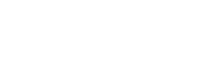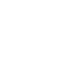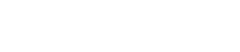## MOKASA Joint Evaluation Examination Mathematics Paper 1 2016- Pre MOCK

SECTION A (50 MARKS)
Answer all questions in this section in the spaces provided

1. Simplify without using table or a calculator to its lowest form. (3 marks)2. Abraham’s money box contains only sh. 5 coins and sh. 10 coins. There are 24 coins and their total value is sh. 150. Find how many of each kind of coins there are in the box. (3 marks)

3. The gradient of a straight line M1 passing through the points (P(3, 4) and Q(x, y) is -3/2. A line M2 is perpendicular to M1 and passes through the points Q and R(2, -1). Determine the values of X and Y. (3 marks)

4. Three inequalities define the region R shown below. Form the inequalities. (3 marks)5. Given that a = 10.5, b = 9.6 and c = 1.4 all correct to the decimal place. Find the percentage error involved in the calculation of (a+b)/to 1 d.p . (3 marks)

6. The GCD of three numbers is 4 while the LCM is 360. If two of the numbers are 24 and 40. List two other possible numbers. (3 marks)

7. Given that dy/dx = 2x3 + 3 and that y = 3 when x = 0, find the value of y when x = 1/3. (3 marks)

8. After buying 52 Sterling pounds, a businessman decided to exchange his money for US dollars. Using the following currency exchange rate, calculate to 3s.f. the number of dollars he ended up with.
1 US dollars (\$) = ksh. 89.75
1 Sterling pound (£) = ksh. 135.47 (3 marks)

9. Solve for X and Y in (3 marks)

4x x 4y = 1
32x-y = 81

10. Given the number 11055. Show that the number is divisible by 3, 5 and 11 using necessary divisibility tests. (3 marks)

11. Calculate the area of the shaded region in the figure below, given that; OX = OY = 10cm and XY = 16 cm. (Take π = 3.142) (4 marks)12. The figure below represents a model of a prism ABCDEF drawn to scale. Complete the prism. (3 marks)13. Use reciprocal table to find the value of 1/0.325. Hence evaluate. (3 marks)

14. A poultry farmer has brown and white chicken; the brown chicken are twice as many as the white ones; of the white chicken 2/5 are layers while the rest are cockerels; of the brown ones 5/12 are layers while the rest are cockerels. If the chicken is picked at random in the dark, find the probability that it will be a cockerel. (3 marks)

15. Triangle PQR has vertices P(3, 2), Q(-1, 1) and R(-3, -1). Under a rotation the vertices of PIQIRI are PI(1, 4), QI(2, 0) and RI(4, -1). By construction find the centre and angle of rotation. (4 marks)16. A metal cuboid of length 12cm, width 10cm and height 6cm has a density of 1.4g per cm3. A cylinder of different metal has a base radius of 6cm and height 12m, with a density of 1.8 g/cm3. The two solids are melted down to recast in the shape of a cube without loss of metal. (Take π=3.142). Calculate the average density of the cube. (3 marks)

SECTION B (50 MARKS)
Answer any five questions in this section

17. Three hundred and sixty litres of a homogenous paint is made by mixing three types of paints A, B and C. The ratio by volume of paint A to paint B is 3:2 and paint B to paint C is 1:2. Paint A costs shs. 180 per litre, paint B shs. 240 per litre and paint C shs. 127.50 per litre and paint C shs. 127.50 per litre.

1. The volume of each type of paint in the mixture. (5 marks)
2. The amount of money spent in making one litre of the mixture. (3 marks)
3. The percentage profit made by selling the mixture at shs. 221 per litre. (2 marks)

18. The figure below is a solid frustum of a rectangular base ABCD and top rectangular EFGH. Given that AB = 6cm, AC = 10cm, HF = 5cm, FG = 4cm and CF =25cm.Calculate:
1. The height of the pyramid it was cut from giving your answer to the nearest whole number. (2 marks)
2. The surface area of the frustum. (5 marks)
3. The volume of the frustum. (3 marks)

1. Complete the table below for the function y = 1/2 sin 2x where 0ox≤360o. (2 marks)2. On the grid provided, draw the graph of the function y = 1/2 sin 2x for 0ox≤360o using the scale 1 cm for 30o on the horizontal axis and 4cm for 1 unit of y-axis. (3 marks)3. Use your graph to determine the amplitude and period of the function y = 1/2 sin 2x. (2 marks)

4. Use the graph to solve;
1. 1/2 sin 2x = 0 (1 mark)
2. 1/2 sin 2x - 0.5 = 0 (2 marks)

19. Using a ruler and a pair of compass only; Construct rectangle ABCD whose sides are AB = 10cm and BC = 7cm.
Use the figure to:
1. Find the point R and S on AD and DC respectively, such that R is equidistant from AD and S is equidistant from DC. (2 marks)
2. Shade the region within the rectangle in which a variable point X must lie given that X satisfies the following conditions.
1. X >2 cm from RS (3 marks)
2. X is nearer to AB than to BC (3 marks)
3. BX is more than 5cm (2 marks)

20. A youth group decided to raise ksh. 480,000 to buy a piece of land costing ksh. 80,000 per hectare. Before the actual payment was made, four of the members pulled out and each of those remaining had to pay an additional ksh. 20,000.
1. If the original number of the group members was X, write down;
1. An expression of how much was contributed originally. (1 mark)
2. An expression of how much the remaining members were to contribute after the four pulled out. (1 mark)

2. Determine the number of members who actually contributed towards the purchase of the land. (4 marks)
3. Calculate the ratio of the supposed original contribution for the new contribution. (2 marks)
4. If the land was sub-divided equally, find the size of land each member got. (2 marks)

21. A train moving at 40km/h is moving in the same direction with a truck on a road parallel to the railway line at a speed of 75km/h. The truck takes 1 ¼ min to overtake the train completely.
1. Given that the truck is 5m long. Determine the length of the train in metres. (6 marks)
2. The truck and the train continued moving parallel to each other at the original speeds. Calculate the distance between them after 10 mins 15 sec from the time the truck overtook the train. (2 marks)
3. The truck stopped 50 minutes after overtaking the train. How long did the train take to catch up with the truck? (2 marks)

1. Given that the position vectors of A, B and C are;Find;
1. AC (1 mark)
2. AB (1 mark)
3. BC (1 mark)

2. In the figure below, OR = 5a, OP = 5b, and PQ = 2ab1. Express as simply as possible, vectors OQ and RQ in terms of a and b. (2 marks)
2. Given that line PQ produced meet at X and that PX = k PQ and OX = h OR where k and h are constants; form an equation connecting k, h, a and b. Hence deduce the values of k and h. (5 marks)

22. Mr. Kamau earns a basic salary of ksh. 30,000 per month. He gets medical allowance of ksh. 4000 per month. He occupies a company house for which he pays a nominal rent of ksh. 1000 per month. He enjoys a tax relief of ksh. 600 per month. The following PAYE is in operation.

 Income K£ P.a. Rate of tax in Ksh. (£) 0 – 3600 2 3601 – 7200 3 7201 – 10800 5 10801 – 14400 7 14401 – 18000 9 18001 and over 10

1. Calculate Kamau’s taxable income in Kenya pounds per annum. (3 marks)
2. Calculate Kamau’s PAYE in Kenya shillings per month. (4 marks)
3. In addition to the PAYE the following deductions were made on his salary every month.
1. WCPs at 2% of basic salary
2. NHIF of ksh. 300
3. Cooperatives shares and loan recovery totaling ksh. 2,500 per month.
Calculate Kamau’s net pay in ksh. per month. (3 marks)

#### Download MOKASA Joint Evaluation Examination Mathematics Paper 1 2016- Pre MOCK.

• ✔ To read offline at any time.
• ✔ To Print at your convenience
• ✔ Share Easily with Friends / Students